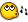## Recommended Posts

I tried looking through the help file but couldnt find the command.

How is it that you compare some numbers and see wich of them that are closest to \$x?

Like in a array or so?

[center][u]WoW Machinima Tool[/u] (Tool for Machinima Artists) [/center]

##### Share on other sites

go through the array and do

\$x - element value

then use

Abs ( expression )

store the element number if smaller than previous element or continue if larger. hope that made sense

##### Share on other sites

I would do this for you but iam feeling lazy.##### Share on other sites

go through the array and do

\$x - element value

then use

Abs ( expression )

store the element number if smaller than previous element or continue if larger. hope that made sense

Hmm.. what would Abs help here?

isnt there a specified command for this?

[center][u]WoW Machinima Tool[/u] (Tool for Machinima Artists) [/center]

##### Share on other sites

This is a quickie that returns "1" if the first one is closer, "2" id the second is closer, and "0"if they are equal distance in comparison to the third number

This example will return a 1 for example

```\$msg = _Compare(100, 200, 0)
MsgBox(0,"", \$msg)

;----------
Func _Compare(\$Number1, \$Number2, \$NumToComp)
Local \$1 = Abs(\$NumToComp - \$Number1)
Local \$2 = Abs(\$NumToComp - \$Number2)
Select
Case \$1 = \$2
\$value = 0
Case \$1 < \$2
\$value = 1
Case \$1 > \$2
\$Value = 2
EndSelect
Return \$Value
EndFunc```
Edited by Paulie

##### Share on other sites

I took the time to write it for youboth functions _ArrayMinDiff and _ArrayMaxDiff return an array with 2 values

elment

 gives the index at which the min difference or max difference was found and

 gives the min or max difference

```\$Array = StringSplit("4,2,6,8,12,5",",")
\$min=_ArrayMinDiff(\$Array,6,1)
\$max=_ArrayMaxDiff(\$Array,6,1)
ConsoleWrite('min:'&\$min&@CRLF&\$min&@CRLF&"max:"&\$max&@CRLF&\$max&@CRLF)

Func _ArrayMinDiff(\$F_Array,\$F_Value,\$i_Base=0)

If Not IsArray(\$F_Array) Then
SetError(1)
Return ""
EndIf

Local \$ArrayMinDiff,\$i,\$i_DiffValue
\$ArrayMinDiff=\$i_Base
Local \$i_Upper = UBound(\$F_Array)-1

For \$i= \$i_Base To \$i_Upper

\$i_DiffValue=Abs(Number(\$F_Array[\$i])-\$F_Value)
if \$i_DiffValue<Abs(Number(\$F_Array[\$ArrayMinDiff])-\$F_Value) then
\$ArrayMinDiff=\$i
\$ArrayMinDiff=\$i_DiffValue
EndIf
Next

SetError(0)
Return \$ArrayMinDiff
EndFunc

Func _ArrayMaxDiff(\$F_Array,\$F_Value,\$i_Base=0)

If Not IsArray(\$F_Array) Then
SetError(1)
Return ""
EndIf

Local \$ArrayMaxDiff,\$i,\$i_DiffValue
\$ArrayMaxDiff=\$i_Base
Local \$i_Upper = UBound(\$F_Array)-1

For \$i= \$i_Base To \$i_Upper

\$i_DiffValue=Abs(Number(\$F_Array[\$i])-\$F_Value)
if \$i_DiffValue>Abs(Number(\$F_Array[\$ArrayMaxDiff])-\$F_Value) then
\$ArrayMaxDiff=\$i
\$ArrayMaxDiff=\$i_DiffValue
EndIf

Next

SetError(0)
Return \$ArrayMaxDiff
EndFunc```
Edited by Cue

##### Share on other sites

Hmm... just one question what exactly is alpha Numeric

##### Share on other sites

I took the time to write it for youboth functions _ArrayMinDiff and _ArrayMaxDiff return an array with 2 values

elment

 gives the index at which the min difference or max difference was found and

 gives the min or max difference

```\$Array = StringSplit("4,2,6,8,12,5",",")
\$min=_ArrayMinDiff(\$Array,6,1)
\$max=_ArrayMaxDiff(\$Array,6,1)
ConsoleWrite('min:'&\$min&@CRLF&\$min&@CRLF&"max:"&\$max&@CRLF&\$max&@CRLF)

Func _ArrayMinDiff(\$F_Array,\$F_Value,\$i_Base=0)

If Not IsArray(\$F_Array) Then
SetError(1)
Return ""
EndIf

Local \$ArrayMinDiff,\$i,\$i_DiffValue
\$ArrayMinDiff=\$i_Base
Local \$i_Upper = UBound(\$F_Array)-1

For \$i= \$i_Base To \$i_Upper

\$i_DiffValue=Abs(Number(\$F_Array[\$i])-\$F_Value)
if \$i_DiffValue<Abs(Number(\$F_Array[\$ArrayMinDiff])-\$F_Value) then
\$ArrayMinDiff=\$i
\$ArrayMinDiff=\$i_DiffValue
EndIf
Next

SetError(0)
Return \$ArrayMinDiff
EndFunc

Func _ArrayMaxDiff(\$F_Array,\$F_Value,\$i_Base=0)

If Not IsArray(\$F_Array) Then
SetError(1)
Return ""
EndIf

Local \$ArrayMaxDiff,\$i,\$i_DiffValue
\$ArrayMaxDiff=\$i_Base
Local \$i_Upper = UBound(\$F_Array)-1

For \$i= \$i_Base To \$i_Upper

\$i_DiffValue=Abs(Number(\$F_Array[\$i])-\$F_Value)
if \$i_DiffValue>Abs(Number(\$F_Array[\$ArrayMaxDiff])-\$F_Value) then
\$ArrayMaxDiff=\$i
\$ArrayMaxDiff=\$i_DiffValue
EndIf

Next

SetError(0)
Return \$ArrayMaxDiff
EndFunc```

Ohhh, you didnt really need to do all that for me, since it was what i tried to avoid.

Im kinda a "tiny code hugger".

[center][u]WoW Machinima Tool[/u] (Tool for Machinima Artists) [/center]

##### Share on other sites

Your welcome. it may come in handy for me in the future aswell## Create an account

Register a new account

• ### Recently Browsing   0 members

×

• Wiki

• Back

• #### Beta

• Git
• FAQ
• Our Picks
×
• Create New...